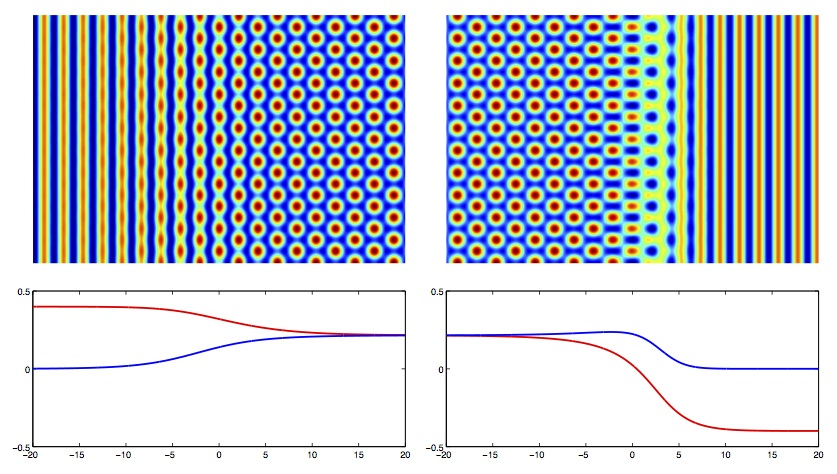### Stationary coexistence of hexagons and rolls via rigorous computations

J.B. van den Berg, A. Deschênes, J.-P. Lessard and J.D. Mireles James

In this work we introduce a rigorous computational method for finding heteroclinic solutions of a system of two second order differential equations. These solutions correspond to standing waves between rolls and hexagonal patterns of a two-dimensional pattern formation PDE model. After reformulating the problem as a projected boundary value problem (BVP) with boundaries in the stable/unstable manifolds, we compute the local manifolds using the Parameterization Method and solve the BVP using Chebyshev series and the radii polynomial approach. Our results settle a conjecture by Doelman et al. [European J. Appl. Math., 14 (1), 85-110, 2003] about the coexistence of hexagons and rolls.

A preprint in its pdf form can be found here.

Here is the MATLAB code which perform the proof of Theorem 1. The code requires activating the interval arithmetic package INTLAB.